# The miracle of the bootstrap

In my opinion one of the most useful tools in the statistician’s toolbox is the bootstrap. Let’s suppose that we want to estimate something slightly non-standard. We have written a program in our favourite statistical package to calculate the estimate. But in addition to the estimate itself, we need a measure of its precision, as given by its standard error. We saw in an earlier post how the standard error can be calculated for the sample mean. With a non-standard estimator, it may too difficult to derive an analytical expression for an estimate of the standard error. Or in some situations it may not be worth the intellectual effort of working out an analytical standard error.

The bootstrap can help us in these settings. The bootstrap is a computational resampling technique for finding standard errors (and in fact other things such as confidence intervals), with the only input being the procedure for calculating the estimate (or estimator) of interest on a sample of data. The idea of the bootstrap is to mimic the process of randomly sampling from an assumed infinite population. Ordinarily, we take a sample from a population, and the standard error reflects the variability between the estimates we would obtain if we repeatedly took samples from the population. The bootstrap mimics this process by treating our observed sample as if it were the population. It then repeatedly takes (say B) samples (of the same size as the original sample), with replacement, from our original sample (note there are other types of bootstrap sampling. This is so called non-parametric bootstrap sampling). For each of these B samples, we then calculate our estimate of interest. We can then use the sample standard deviation of these estimates, across bootstraps, as an estimate of standard error. Let’s express this algebraically.

Let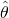denote the estimate of our parameter (what it is), from the original sample. Then let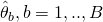denote the B estimates of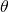from the bootstrap samples. The bootstrap standard error foris then given by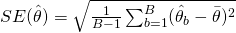where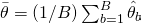denotes the mean of the estimates across the B bootstrap samples.

The ‘miracle’ part is that we have to do no hard maths to come up with an analytical formula for the standard error, based on our particular estimator. As mentioned previously, this means it is particularly attractive when finding a standard error is difficult.

Furthermore, because the only input is the procedure for calculating the estimate of interest, it is easy to bootstrap your procedure in statistical software. In R you can use the boot package and in Stata you can prefix many built in commands, and your own user-written commands, with the bootstrap command. These packages also calculate various bootstrap confidence intervals. Some of these (e.g. bias corrected and accelerated) are particularly useful when the sampling distribution of the estimator is far from normal, such that forming a symmetric Wald type confidence interval would be inappropriate.

The boot package in R
To illustrate the simplicity of the bootstrap we will use it to give us a standard error for a very simple situation, in which we actually know how to calculate a standard error analytically. This way we can compare the result we get from the bootstrap with the one from the analytical formula. Of course typically we will be using the bootstrap in situations where we do not have an analytical standard error available.

We will simulate 100 random observations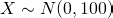. Now the standard error for the sample mean is given by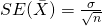where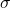denotes the standard deviation of the variable in the population and n is the sample size. Of course we do not knowtypically, so we substitute an estimate of it, i.e. the sample SD: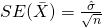In our artifical example, we are simulating X with a variance of 100, and so a standard deviation of 10. If we take a sample of size n=100, the true standard error, which measures variability in the sample mean in repeated samples, is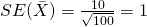To simulate the data in R we can use the following code:

set.seed(61231601)
n <- 100
sigma <- 10
x <- sigma*rnorm(n)


We can calculate an estimated standard error from the sample data by:

sd(x)/10
 0.9800282


which is close to the standard error of 1. Now let's see how the bootstrap can give us a standard error without having to know the formula for the standard error of the mean. First you need to make sure you have installed the boot package into R, and loaded it:

library(boot)


Before we perform the bootstrap, we will define a slightly modified version of R's mean function:

bootmean <- function(d, i) mean(d[i])


This modified mean function, which I've called bootmean, takes two arguments. The first is the data vector, which I've labelled d here. The second argument, which I've called i, will be a vector of indices. This is needed because the boot function will pass us a vector of indices which indicates the indices of the observations which have been sampled, when it performs its sampling with replacement. So, if observation 1 is selected twice, the index 1 will appear twice in i. The bootmean function then simply returns the mean of d, but where we use the vector i to select which observations have been selected (note that observations can be selected more than once because bootstrap sampling is with replacement).

Now we call the boot function:

bs <- boot(x, bootmean, R=1000, stype="i")
print(bs)

ORDINARY NONPARAMETRIC BOOTSTRAP

Call:
boot(data = x, statistic = bootmean, R = 1000, stype = "i")

Bootstrap Statistics :
original      bias    std. error
t1* 0.8863115 -0.01071582   0.9722003


The first argument we pass to boot is our original data vector, x. The second argument is the function which calculates our statistic of interest, which here is our modified bootmean function. The third argument specifies that we want 1,000 bootstrap samples to be used. The final argument specifies what our user defined function (i.e. bootmean) is expecting in its second argument. Because we have defined bootmean to expect a vector of indices, we specify stype="i" (i for indices).

The output we receive gives us value of a our function (bootmean) applied to our original data, which is just the original sample mean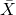. The next number is the bootstrap estimate of bias (which we've not gone into here), and lastly the bootstrap estimated standard error, which here is 0.972. This is remarkably close to the analytical standard error of 0.980. So, the bootstrap has given us standard error without requiring us to work out an analytical formula for the standard error.

Try running the last two lines of code a second time. You should obtain a different bootstrap standard error value. This is because the boot command takes another 1,000 bootstrap samples of the original data, which will not be the same as the original 1,000, and so obtains a slightly different standard error. This difference is usually referred to as Monte-Carlo error. We can make it arbitrarily small but using a larger number of bootstrap replicates.

Earlier we mentioned that bootstrap can also be used to find confidence intervals. To do this we simply type:

> boot.ci(bs)
BOOTSTRAP CONFIDENCE INTERVAL CALCULATIONS
Based on 1000 bootstrap replicates

CALL :
boot.ci(boot.out = bs)

Intervals :
Level      Normal              Basic
95%   (-1.0085,  2.8025 )   (-0.9677,  2.7448 )

Level     Percentile            BCa
95%   (-0.9721,  2.7404 )   (-0.8243,  2.8623 )
Calculations and Intervals on Original Scale
Warning message:
In boot.ci(bs) : bootstrap variances needed for studentized intervals


This gives us a number of different confidence intervals for the population mean. The normal one is found by subtracting/adding 1.96 times the bootstrap the standard error to the sample mean, which would be a valid way to construct a confidence interval if the sampling distribution of the sample mean were normal (here it is, but in other situations it might not be). In fact, the 'normal' CI calculated by the boot function adjusts the lower and upper limits by the bootstrap estimate of bias as well.

The other intervals are different approaches, but they are all based on the bootstrap distribution of the sample means. For example, the percentile methods takes the 2.5% and 97.% centiles of the bootstrap sample means to obtain the lower and upper limits. The bias corrected and accelerated (BCa) method is a more elaborate version, which has a number of more theoretical advantages compared to the percentile interval.

Books on the bootstrap
My personal favourite text on the bootstrap is Efron and Tibshirani's An Introduction to the Bootstrap. As well as explaining the bootstrap, this book also contains very nice material on statistical inference more generally. The other main text is Davison and Hinkley's Bootstrap Methods and their Application.

### 2 thoughts on “The miracle of the bootstrap”

1.Shouldn’t the bootstrap standard error be the standard deviation, rather than the variance, of the individual bootstrap estimates? I think you’re equation is missing a square root.

•